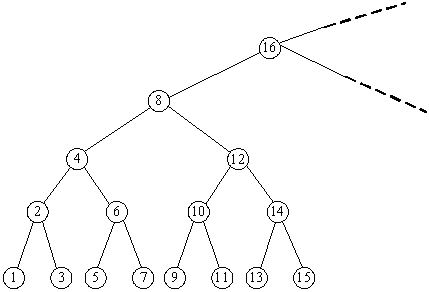# POJ 2309 BST

Consider an infinite full binary search tree (see the figure below), the numbers in the nodes are 1, 2, 3, …. In a subtree whose root node is X, we can get the minimum number in this subtree by repeating going down the left node until the last level, and we can also find the maximum number by going down the right node. Now you are given some queries as “What are the minimum and maximum numbers in the subtree whose root node is X?” Please try to find answers for there queries.Input

In the input, the first line contains an integer N, which represents the number of queries. In the next N lines, each contains a number representing a subtree with root number X (1 <= X <= 231 – 1).

Output

There are N lines in total, the i-th of which contains the answer for the i-th query.

Sample Input

Sample Output